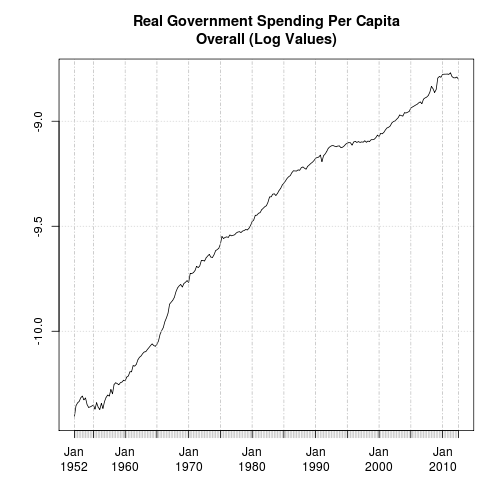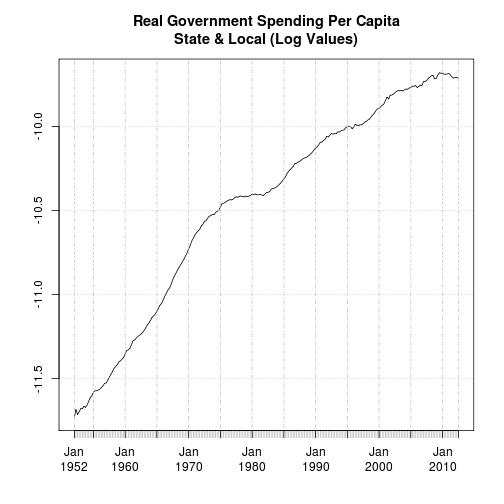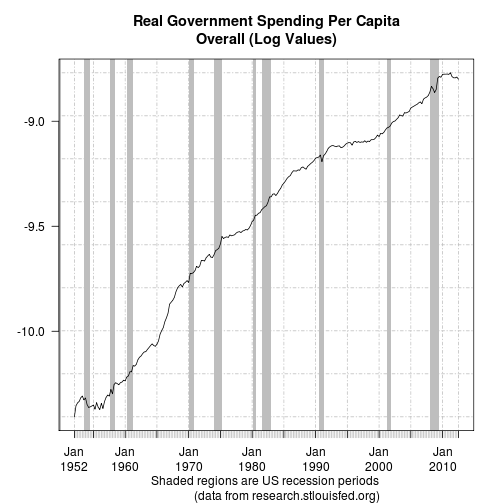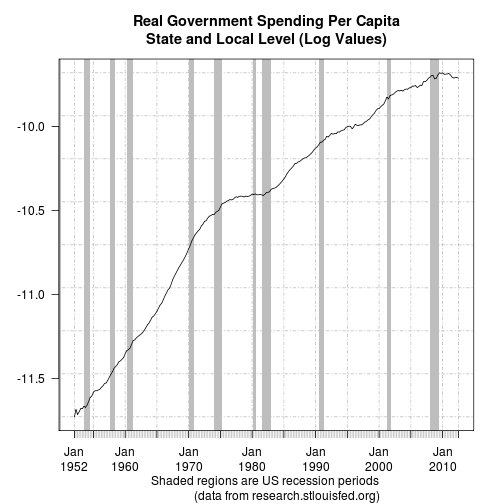## 2012-10-27

### Replicating Krugman (Pt. 1)

I'm a regular reader of Paul Krugman's NYT blog. A couple of months ago I decided to replicate some of the plots Paul published. At the time I was evaluating the quantmod package which seemed to made things easy.

## An easy start

In the first part I'll reproduce two plots from Real Government Spending Per Capita. It's straightforward to get the data using quantmod:

``````require(quantmod)

# First we get the time series from the Federal Reserve Bank of St. Louis (FRED)
getSymbols('GEXPND', src = 'FRED')
getSymbols('GDPDEF', src = 'FRED')
getSymbols('SLEXPND', src = 'FRED')
getSymbols('POP', src = 'FRED')
getSymbols('USREC', src = 'FRED')

# Paul Krugman doesn't use the raw series but real per capita values.
# These are the necessary calculations.
log_gov_overall <- log(GEXPND/(GDPDEF*POP), exp(1))
log_gov_state_local <- log(SLEXPND/(GDPDEF*POP), exp(1))
``````
It's just as easy to create the standard plots:
``````plot(log_gov_overall
, main = "Real Government Spending Per Capita\nOverall (Log Values)"
)
````````````plot(log_gov_state_local
, main = "Real Government Spending Per Capita\nState & Local (Log Values)"
)
``````A closer look, however, reveals that something is clearly missing. Paul took his plots straight from FRED itself. One of the features is that recessions are shown by graying the background.

## FRED style plots

After struggling with the parameters and different quantmod plots for a while I decided to write a function that takes the series to be plotted as a parameter and creates a FRED style plot.

This makes some use of the internal workings of plot.xts:
``````FREDplot <-  function (x
, main = deparse(substitute(x))
, las = 1
, ...) {
# function to create FRED style plots with shaded regions for U.S. recessions
# this function needs the time series to be xts
if  (!(class(x) == "xts") &&
(class(x) == "zoo")){
stop("Only xts objects can be properly handled by this function!")
}
# get the recession dates from FRED only once
if  (!("USREC" %in% ls(name = globalenv()) &&
attributes(USREC)\$src == "FRED")){
getSymbols('USREC', src = 'FRED')
}

# transform FRED recession dates to xy.coordinates
usrec <- xy.coords(.index(USREC), USREC[, 1])

# prepare plot
xycoords <- xy.coords(.index(x), x[, 1])
ep <- axTicksByTime(x, TRUE, format.labels = TRUE)
plot.zoo(xycoords\$x, xycoords\$y
, type = 'n', axes = FALSE, ann = FALSE
)

# plot axis & box
axis(1, at = xycoords\$x, labels = FALSE
, col = "#BBBBBB"
)

axis(1, at = xycoords\$x[ep], labels = names(ep)
, las = 1,lwd = 1, mgp = c(3, 2, 0)
)

axis(2, las = las)

xblocks(usrec\$x,ifelse(usrec\$y == 1, "gray", "white"))

abline(v = xycoords\$x[ep], col = "grey", lty = 4)
abline(h = seq(min(xycoords\$y), max(xycoords\$y)
, length.out = 9), col = "grey", lty = 4
)

# plot curve
lines(xycoords\$x, xycoords\$y,...)

title(main = main)
title(sub = "Shaded regions are US recession periods
(data from research.stlouisfed.org)"
)

box()
}

``````
Using this function the plots look much better:
``````# the first plot
FREDplot(log_gov_overall,
main = "Real Government Spending Per Capita\nOverall (Log Values)")
````````````
# and the second
FREDplot(log_gov_state_local,
main = "Real Government Spending Per Capita\nState and Local Level (Log Values)")
``````Actually I like these plots better than the originals here and there.
There are, however, a couple of things that could - and should - be improved. More of this in future installments.
I'll call it a day for now.